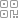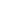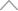6 0 8 4 5 1 9

Python Bokeh 库进行数据可视化实用指南写在前面什么是Bokeh？

Bokeh 是 Python 中的交互式可视化库。Bokeh提供的最佳功能是针对现代 Web 浏览器进行演示的高度交互式图形和绘图。Bokeh 帮助我们制作出优雅、简洁的图表，其中包含各种图表。Bokeh 主要侧重于将数据源转换为 JSON 格式，然后用作 BokehJS 的输入。Bokeh的一些最佳功能是：

• 灵活性：  Bokeh 也为复杂的用例提供简单的图表和海关图表。

• 功能强：  Bokeh 具有易于兼容的特性，可以与 Pandas 和 Jupyter 笔记本一起使用。

• 样式：  我们可以控制图表，我们可以使用自定义 Javascript 轻松修改图表。

• 开源：  Bokeh 提供了大量的示例和想法，并在 Berkeley Source Distribution (BSD) 许可下分发。

在哪使用Bokeh图

• Bokeh模型

• Bokeh图

• Bokeh应用

• Bokeh服务器

Bokeh模型

Bokeh模型提供低级接口，为应用程序开发人员提供高端灵活性

Bokeh图

Bokeh绘图提供了一个用于创建视觉符号的高级界面。Bokeh绘图是 Bokeh.models 模块的子类。它包含 图形 类的定义；图形类是最简单的绘图创建。

Bokeh应用程序

Bokeh应用程序包，用于创建Bokeh文件；是一家轻量级工厂。

Bokeh服务器

Bokeh 服务器用于发布和共享交互式图表和应用程序。

安装Bokeh库

pip install pandas-Bokeh

conda install -c patrikhlobil pandas-Bokeh

导入Bokeh库

import pandas as pd

# pip install pandas_Bokeh

import pandas_Bokeh

from Bokeh.io import show, output_notebook

from Bokeh.plotting import figure

pandas_Bokeh.output_notebook()

pd.set_option('plotting.backend''pandas_Bokeh')

Bokeh绘图是一个用于创建交互式视觉效果的界面，我们从中导入 它作为保存我们图表的容器。

figure

from Bokeh.plotting import figure

from Bokeh.io import show, output_notebook

pandas_Bokeh.output_notebook()

pandas_bokeh.output_file（文件名）

Hovertool 用于在我们使用鼠标指针悬停在数据上时显示值， ColumnDataSource 是 DataFrame 的 Bokeh 版本。

from Bokeh.models import HoverTool, ColumnDataSource

绘制图表的语法

dataframe.plot_Bokeh()

fig = figure()

'''

'''

show(fig)

• x  – 包含图表 x 轴数据的 ColumnDataSource 列的名称

• y  – 包含图表 y 轴数据的 ColumnDataSource 列的名称

• source  – ColumnDataSource 列的名称，该列包含我们为 x 轴和 y 轴引用的数据

output_file('abc.html')

使用Bokeh库主题

Bokeh主题有一组预定义的设计，可以将它们应用到您的绘图中。Bokeh 提供了五个内置主题。

• caliber,

• dark_minimal,

• light_minimal,

• night_sky,

• contrast.图表样式

• 线属性

• 填充属性

• 文本属性

fig = figure(background_fill_color="#fafafa")

fig = figure(height=350, width=500)

fig.axis.visible=False

fig.grid.grid_line_color = None

fig.background_fill_alpha=0.3

fig = figure(title="abc")

fig.xaxis.axis_label='X-axis'

fig.yaxis.axis_label='Y-axis'

简单样式的演示图表

x = list(range(11))

y0 = x

fig = figure(width=500, height=250,title='Title',background_fill_color="#fafafa")

fig.circle(x, y0, size=12, color="#53777a", alpha=0.8)

fig.grid.grid_line_color = None

fig.xaxis.axis_label='X-axis'

fig.yaxis.axis_label='Y-axis'

show(fig)• 准备数据

• 创建一个新的情节

• 为您的数据添加渲染，以及您对绘图的可视化自定义

• 指定生成输出的位置（在 HTML 文件中或在 Jupyter Notebook 中）

• 显示结果

Python 中的Bokeh用例

数据

import glob

path = 'archive'

all_files = glob.glob(path + "/*.csv")

li = []

usr=0

for filename in all_files:

usr+=1

df['User ID']=usr

li.append(df)

df = pd.concat(li, axis=0, ignore_index=True)

df[:2]数据说明

• Game Completed Date -游戏完成的日期和时间

• Team团队 - 告诉我们玩家是冒名顶替者还是船员

• Outcome结果 - 告诉我们游戏是否赢/输

• Task Completed已完成 的任务 - 船员完成的任务数

• All Tasks Completed  – 布尔变量显示所有任务是否由船员完成

• Murdered谋杀 - 船员是否被谋杀

• Imposter Kills冒名顶替者杀死  – 冒名顶替者的击杀次数

• Game Length游戏时长 ——游戏的总持续时间

• Ejected  - 玩家是否被其他玩家驱逐

• Sabotages Fixed  – 船员修复的破坏次数

• Time to complete all tasks完成所有任务的 时间——船员完成任务所用的时间

• Rank Change排名变化 - 比赛输/赢后排名的变化

• Region/Game Code地区/游戏代码 - 服务器和游戏代码

• User ID用户 ID  – 用户 数量。

df.describe(include='O')df['Min'] = df.apply(lambda x : x['Game Length'].split(" ") , axis = 1)

df['Min'] = df['Min'].replace('m''', regex=True)

df['Min'][:2]

0 07

1 16

Name: Min, dtype: object

df['Murdered'].replace(['No''Yes''-'], ['Not Murdered''Murdered''Missing'],inplace=True)

饼形图

df_team = df.Team.value_counts()

df_team.plot_Bokeh(kind='pie', title='Ration of Mposter vs Crewmate')79%

，Imposters 占

21%

，由此可见

Imposter: Crewmates

的比例为

1:4

。冒名顶替者较少，因此有可能赢得大部分比赛。

圆环图

from math import pi

df_mur = df.Murdered.value_counts().reset_index().rename(columns={'index''Murdered''Murdered''Value'})

df_mur['Angle'] = df_mur['Value']/df_mur['Value'].sum() * 2*pi

df_mur['Color'] = ['#3182bd''#6baed6''#9ecae1']

df_murannular_wedge()

from Bokeh.transform import cumsum

fig = figure(plot_height=350

title="Ration of Murdered vs Not Murdered"

toolbar_location=None,

tools="hover", tooltips="@Murdered: @Value", x_range=(-.5.5))

start_angle=cumsum('Angle', include_zero=True),

end_angle=cumsum('Angle'),

line_color="white", fill_color='Color', legend_label='Murdered', source=df_mur)

fig.axis.axis_label=None

fig.axis.visible=False

fig.grid.grid_line_color = None

show(fig)Bokeh圆环图

散点图

df_min = pd.crosstab(df['Min'], df['Sabotages Fixed']).reset_index()

df_min = df_min.rename(columns={0.0:'0T'1.0:'1T',

2.0:'2T',3.0:'3T',4.0:'4T',5.0:'5T'

})

df_min[:2]0,1 和 2

并创建一个数据框。

df_0 = df_min[['Min''0T']]

df_1 = df_min[['Min''1T']]

df_2 = df_min[['Min''2T']]

scatter()

df_min.plot_Bokeh.scatter(x='Min', y='1T')Bokeh散点图

fig = figure(title='Sabotages Fixed vs Minutes'

tools= 'hover'

toolbar_location="above"

toolbar_sticky=False)

fig.circle(x="Min",y='0T'

size=12, alpha=0.5

color="#F78888"

legend_label='0T'

source=df_0),

fig.circle(x="Min",y='1T'

size=12, alpha=0.5

color="blue"

legend_label='1T'

source=df_1),

fig.circle(x="Min",y='2T'

size=12, alpha=0.5

color="#626262"

legend_label='2T'

source=df_2),

show(fig)Bokeh散点图

简单直方图

hist

df_minutes = df['Min'].astype('int64')

df_minutes.plot_Bokeh(kind='hist', title='Distribution of Minutes')Bokeh中的直方图

堆积直方图

hist

df_gm_te = pd.crosstab(df['Game Length'], df['Team'])

df_gm_tedf_gm_te.plot_Bokeh.hist(title='Gamelegth vs Imposter/Crewmate', figsize=(750350))Bokeh中的堆叠直方图

不同类型的条形图

简单条形图

df_tc.plot_Bokeh(kind='bar', y='Count', title='How many people have completed given task?', figsize=(750350))Bokeh中的条形图

堆积条形图

df1 = pd.crosstab(df['Team'], df['Outcome'])

df1.plot_Bokeh.bar(title='Who wins: Imposter or Crewmates',stacked=True,

figsize=(550350))Bokeh中的堆积条形图

堆积垂直条形图

df2 = pd.crosstab(df['Outcome'], df['All Tasks Completed'])

df2.plot_Bokeh.barh(title='Completeing task: win or loss', stacked=True,

figsize=(650350))Bokeh中的堆积条形图

双向条形图

df_user = pd.crosstab(df['User ID'], df['Outcome']).reset_index()

df_user['Loss'] = df_user['Loss']*-1

df_user['User ID'] = (df_user.index+1).astype(str) + ' User'

df_user = df_user.set_index('User ID')

df_user[:2]

barh()

在两个方向上制作一个条形图即可。

df_user.plot_Bokeh.barh(title='Users: Won or Defeat')Bokeh中的双向条形图

折线图

line

用来制作折线图。

df_crewmate = df[df['Team'] == 'Crewmate']

df_t_ej = pd.crosstab(df_crewmate['User ID'], df_crewmate['Ejected']).reset_index()

df_t_ej = df_t_ej[['No','Yes']]

df_t_ej.plot_Bokeh.line(title='Cremates Memebers: Ejected vs Minutes', figsize=(750350))Bokeh中的折线图

棒棒糖图表

df_user_new = pd.crosstab(df['User ID'], df['Outcome']).reset_index().sort_values(by='Win', ascending=False)[:10]

df_user_new['User ID'] = (df_user_new.index+1).astype(str) + ' User'

df_user_new[:2]

x = df_user_new['Win']

factors = df_user_new['User ID'#.values

fig = figure(title="Top 10 Users: Win", toolbar_location=None,tools="hover", tooltips="@x",

y_range=factors, x_range=[0,75],

plot_width=750, plot_height=350)

fig.segment(0, factors, x, factors, line_width=2, line_color="#3182bd")

fig.circle(x, factors, size=15, fill_color="#9ecae1", line_color="#3182bd", line_width=3)

fig.xgrid.grid_line_color = None

fig.ygrid.grid_line_color = None

show(fig)Bokeh中的棒棒糖图

面积图

from Bokeh.models import ColumnDataSource

from Bokeh.plotting import figure, output_file, show

# data

df_min = pd.crosstab(df['Min'], df['Sabotages Fixed']).reset_index()

df_min = df_min.rename(columns={0.0:'0T'1.0:'1T',2.0:'2T',3.0:'3T',4.0:'4T',5.0:'5T'})

# chart

names = ['0T','1T']

source = ColumnDataSource(data=dict(x = df_min.Min,

y0 = df_min['0T'],

y1 = df_min['1T']))

fig = figure(width=400, height=400, title='Sabotages Fied vs Minutes')

fig.varea_stack(['y0','y1'], x='x', color=("grey""lightgrey"),legend_label=names, source=source)

fig.grid.grid_line_color = None

fig.xaxis.axis_label='Minutes'

show(fig)Bokeh中的面积图

Bokeh库的布局功能

Layout 函数将让我们构建一个由绘图和小部件组成的网格。我们可以在一个布局中拥有尽可能多的行和列或网格。

• 如果要 垂直 显示图，请使用**

column()

**函数。

• 如果要 水平 显示图，请使用**

row()

**函数。

• 如果您希望以网格方式绘制图形，请使用**

gridplot()

**函数。

• 如果您希望图表以最佳方式放置，请使用**

layout()

**函数

from Bokeh.io import output_file, show

from Bokeh.layouts import row,column

from Bokeh.plotting import figure

output_file("layout.html")

x = list(range(11))

y0 = x

y1 = [10 - i for i in x]

y2 = [abs(i - 5for i in x]

# create three plots

s1 = figure(width=250, height=250, background_fill_color="#fafafa")

s1.circle(x, y0, size=12, color="#53777a", alpha=0.8)

s2 = figure(width=250, height=250, background_fill_color="#fafafa")

s2.triangle(x, y1, size=12, color="#c02942", alpha=0.8)

s3 = figure(width=250, height=250, background_fill_color="#fafafa")

s3.square(x, y2, size=12, color="#d95b43", alpha=0.8)

column()

函数，输出将如下所示。

show(column(s1, s2, s3))row()

函数，输出将如下所示。

# 将结果排成一行并显示

show(row(s1, s2, s3))layout = grid([[fig1],

[fig2, fig3]])

from Bokeh.io import output_file, show

from Bokeh.plotting import figure

from Bokeh.layouts import column, grid

# 1 layout

df_user_new = pd.crosstab(df['User ID'], df['Outcome']).reset_index().sort_values(by='Win', ascending=False)[:10]

df_user_new['User ID'] = (df_user_new.index+1).astype(str) + ' User'

x = df_user_new['Win']

factors = df_user_new['User ID'

fig1 = figure(title="Top 10 Users: Win", toolbar_location=None,

tools="hover", tooltips="@x",

y_range=factors, x_range=[0,75],

width=700, height=250)

fig1.segment(0, factors, x, factors, line_width=2, line_color="#3182bd")

fig1.circle(x, factors, size=15, fill_color="#9ecae1", line_color="#3182bd", line_width=3)

# 2 layout

df_mur = df.Murdered.value_counts().reset_index().rename(columns={'index''Murdered''Murdered''Value'})

df_mur['Angle'] = df_mur['Value']/df_mur['Value'].sum() * 2*pi

df_mur['Color'] = ['#3182bd''#6baed6''#9ecae1']

fig2 = figure(height=300,width=400, title="Ration of Murdered vs Not Murdered"

toolbar_location=None, tools="hover", tooltips="@Murdered: @Value", x_range=(-.5.5))

start_angle=cumsum('Angle', include_zero=True), end_angle=cumsum('Angle'),

line_color="white", fill_color='Color', legend_label='Murdered', source=df_mur)

# 3 layout

df_team = pd.DataFrame(df.Team.value_counts()).reset_index().rename(columns={'index''Team''Team''Value'})

df_team['Angle'] = df_team['Value']/df_team['Value'].sum() * 2*pi

df_team['Color'] = ['#3182bd''#6baed6']

fig3 = figure(height=300, width=300, title="Ration of Cremates vs Imposter",

toolbar_location=None, tools="hover", tooltips="@Team: @Value", x_range=(-.5.5))

start_angle=cumsum('Angle', include_zero=True), end_angle=cumsum('Angle'),

line_color="white", fill_color='Color', legend_label='Team', source=df_team)

# Styling

for fig in [fig1, fig2, fig3]:

fig.grid.grid_line_color = None

for fig in [fig2, fig3]:

fig.axis.visible=False

fig.axis.axis_label=None

layout = grid([

[fig1],

[fig2, fig3]

])

show(layout)新知精选

IT研发知识▪优秀创作者

优选课程 新知学院

•扫码下载商业新知APP~

•回到顶部